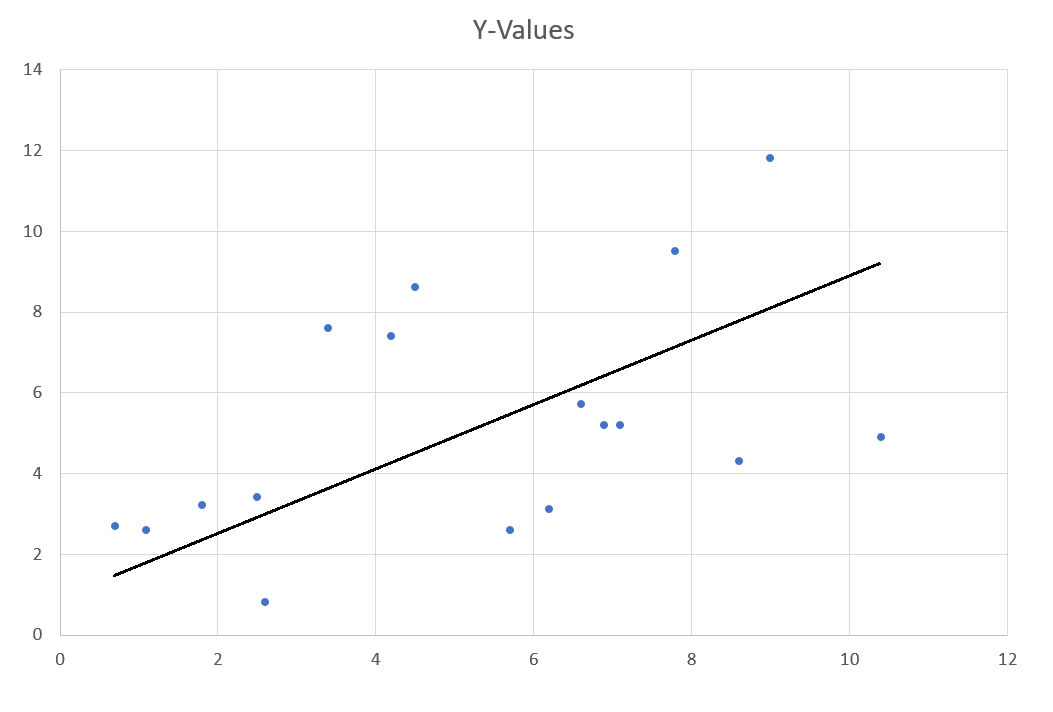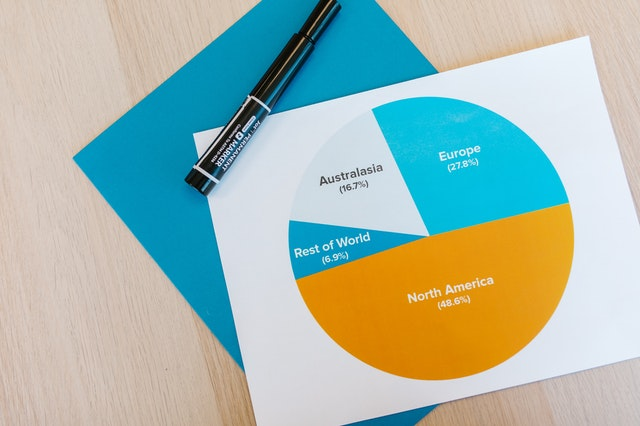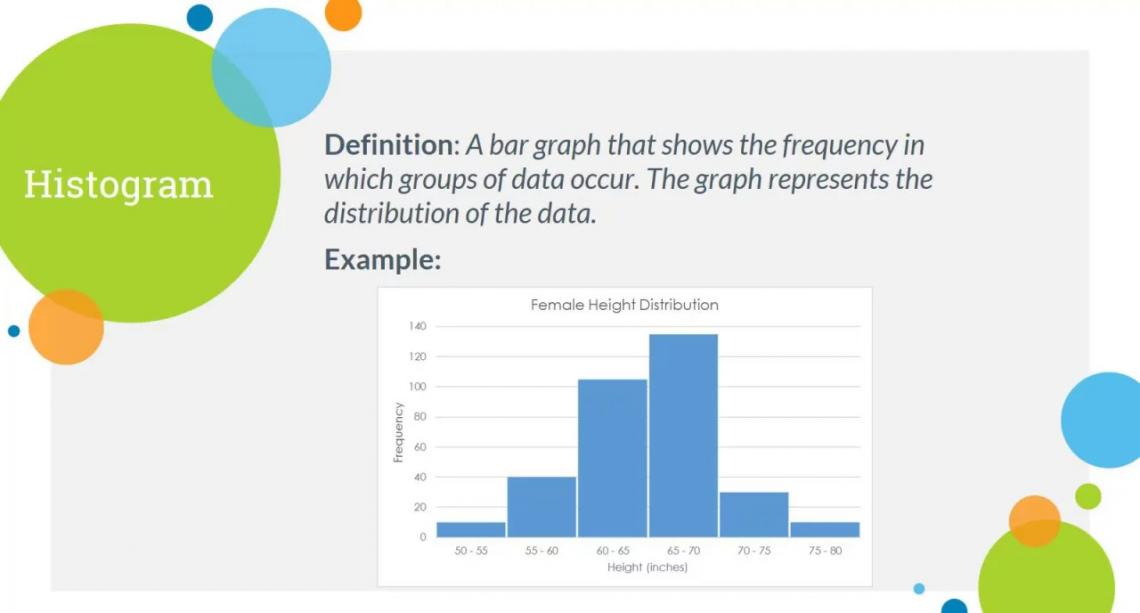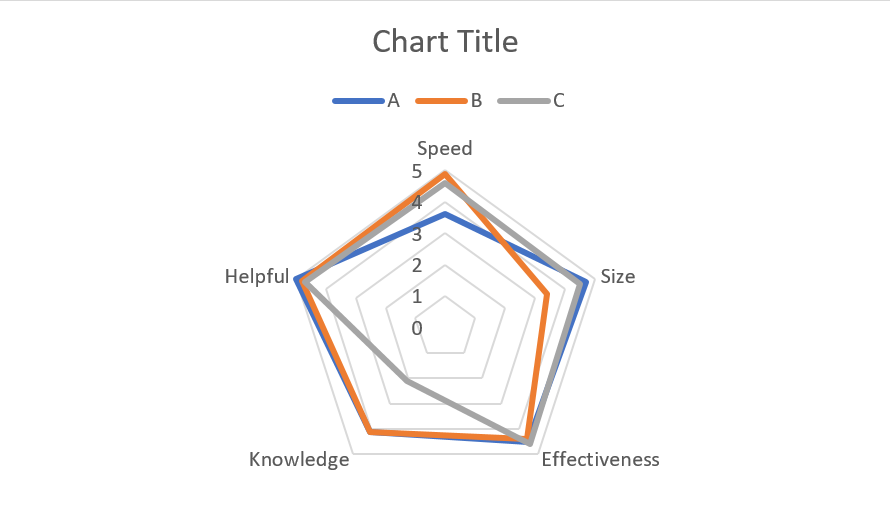# Types of Graphs

List of top 10 types of graphs for dataReviewed by

Patrick Curtis

Expertise: Private Equity | Investment Banking

Updated:

March 9, 2022

A graph is a visual representation of data. They can be static or interactive and may utilize various design strategies to help viewers understand the information being displayed. Therefore, they are useful to communicate complex or large amounts of data in a concise manner.

Hence, keeping a minimalistic look (e.g., not having too many overlapping elements) with 2D (while avoiding 3D) graphs is the best practice. Effective communication of complex facts and figures is a key skill requirement for financial analysts, and thus, extensive knowledge of graphs is a must-have in the finance world.With so many different types of graphs, it can be difficult to figure out which one will work best for your data. In this article, we will go through some of the most common graphs that are used to convey various types of information to make the task easier.

We provide our readers with various templates such as the charts & figures template, which allows them to explore concepts with hands-on experience using these plug-and-play examples. Below, in our line graph section, is a sneak peek of what you can get from this template, allowing you to showcase some of your financial data through graphs and figures.

## 1. Line graph

A line graph is a type of graph that shows a series of data points in the form of a line. These are among the simplest types of graphs and are arguably the easiest to read. It is very useful for displaying changes over time, such as changes in stock prices, where analysts can identify trends. Additionally, you can have multiple lines on a line graph to display different results for comparison without confusing the viewer with too much information. The image from our charts template below is a perfect example of this.To achieve the most aesthetic look for the chart, you can:

• Remove all borders and shading
• Color each line with different shades, with a legend illustrating what each one stands for
• Remove all or most gridlines, as horizontal lines may be useful in reading the graph

Of course, these tips depend on whether the graph is used to supplement a large or decent amount of information, in which case it would be fine to provide an extremely minimalistic graph. However, if the line graph is being used on its own, it would be better to include some more details to not confuse the audience.

Below are the most basic components that are required to be a part of a line graph:

• Title
• The X-axis (horizontal axis)
• The Y-axis (vertical axis)
• Trend / line

## 2. Bar graph

The bar graph is the simplest and most common graph. It is designed to show comparisons between categories and is often used to compare information. Since bar graphs deal with categories, the input data must also be categorical or discrete, meaning whole values. In addition to having categories on the x-axis, there is also a specific type of bar graph called a stacked bar graph, where you can further split each bar into categories.

For example, revenue generated by a company could be separated into cash sales, credit sales, etc. Therefore, one can use a bar graph to dissect complex information into more manageable pieces to compare.Like the line graph, the bar graph has a horizontal (x) axis and a vertical (y) axis. The x-axis represents the independent variable (the variable being changed, like years or units sold), whereas the y-axis represents the dependent variable (the one being measured, like profit).

Data points are plotted on either axis as bars that intersect with both axes at their ends. In other words, this type of graph shows differences between categories by showing parallel lines with lengths proportional to the values being measured.

Bar graphs are useful for showing changes over time because one can easily see how values have increased or decreased over a given period. They are also useful for comparing two sets of data simultaneously.

The aesthetics of bar graphs can be like the line graph in terms of minimalism with borders and grids and the use of a legend.

## 3. Scatter plot

A scatter plot graph is the most straightforward graph. It displays individual data points on a graph that can be linear or nonlinear. Whether a scatter plot is linear or nonlinear simply depends on the shape of the trend created by the data points, with the plot illustrated below being linear.

Scatter plots are useful for showing how two variables are related because it's easy to see clusters of points in different regions of the graph, giving insight into how they are related.The correlation between the variables can be determined by examining the distribution of data points (where they are plotted throughout the graph), where there are clusters of points, and if they are close together or spread out over various regions. The more clustered data is, the higher the correlation between variables, and vice versa. Data points spread out loosely across the graph represent low correlation.

Drawing a line of regression based on this analysis can also help visualize the relationship between variables. A line of regression is a straight line that indicates the trend of the data, which is why it is sometimes called the line of best fit since it can be difficult to have a straight line represent this trend. Having a line of regression signifies a linear relationship as opposed to a nonlinear one.

For example, suppose you want to show the relationship between a person's intelligence level and their age. You could use an interactive scatter plot to display the data collected for both variables from your study participants. If you see a cluster of younger subjects with low intelligence levels and another cluster of older subjects with high intelligence levels, you can surmise that intelligence level increases as people get older and vice versa.

## 4. Pie chart

Pie charts are typically used to show the proportion of a whole, providing a percentage breakdown of a few data points.

For example, if you compare three types of ice cream and want to show the percentage of market share, you would create a pie chart showing that 30% of people prefer chocolate ice cream, 25% like vanilla, and 40% enjoy strawberry. However, if you have data points that are similar in value (e.g., 29% and 30%), the pie chart will not be as useful since they visually look the same, causing confusion amongst readers.Pie charts can become difficult to read if there are too many groups being represented, and thus, it is recommended to cap the number of portions at five. It can be harder to convey information with pie charts due to various reasons, and as such, they are not recommended for financial presentations. These drawbacks include:

• They become cluttered when too many groups are inserted
• It can be harder to identify the variables since there are no axes that tell the reader what data is looked at the relationship between them
• It limits the amount of information you can include due to how easy it can be to complicate a pie chart
• It does not provide a meaningful way to compare information between variables

## 5. Histogram

A histogram is a type of graph that displays the distribution of values in a dataset. It is presented as a bar chart with contiguous bars grouped (no gaps between bars, with only a subtle border to separate them), with the height of each bar representing the frequency or relative frequency of data points in that group as a number or percentage.

Histograms are useful for showing how different categories are distributed across different ranges. For example, you could use a histogram to display the distribution of test scores for students in grades 5-10. The height of each bar would represent how many students scored within that specific range.A cumulative frequency polygon/histogram, or ogive, is essentially a line on the graph from left to right, which adds up the frequency of values for each group, eventually reaching the total value of all groups on the right of the chart. This will be 100% if the unit of measurement is in percentage. It is typically used in conjunction with a histogram and can provide key information, like how many people scored below 50% in a test, for example.

Here is a short video covering the five types of graphs we have gone through:

## 6. Density plot

A density plot is a type of graph that utilizes two variables to display data, usually population or area. The x-axis shows the range of values for one variable, while the y-axis displays the second variable. It is a smoothed version of the histogram, with the frequency of results in the histogram translated to density in a density plot.It will look similar to an area graph but with just one source of input instead of a few sources. The graph's peaks will display where most of the values are concentrated, hence giving the name of the density plot. This article provides a nice, short explanation of this type of graph as well as some nice illustrations like the graph above.

One example might be comparing the number of people in different age ranges. On the x-axis, you would see age ranges from 0-5 years old to 80+ years old. On the y-axis, you would see how many people fall into each range, like 2 million to 12 million people.

An interactive density plot allows viewers to hover over a specific region and view the exact value of the variable at that point. This enables viewers to better understand what exactly they're seeing and where they should focus their attention.

Radar charts/graphs (or spider charts) are a type of circular charts that show data from different angles. It's designed to show how one variable relates to another and is perfect for comparing multiple variables simultaneously.

They are best used for giving scores or ratings to qualitative data, which, in a sense, describes an entity's performance in these fields.For instance, we can use a radar graph to measure multiple aspects of different companies to see what benefits they provide overall.

Like a pie chart, it is very easy to overcomplicate this chart. So, keeping a minimalistic chart with fewer measurement points and factors to compare is the best practice that helps avoid cluttering the chart.

## 8. Area graph

An area graph visually represents the relationship between two variables and multiple input sources being compared (such as the revenue over twelve months of a few companies). A typical example of an area graph is a stacked bar graph, where the height of each bar represents one of two variables. The height for each bar is determined by adding the total number of items in both categories and dividing by the number of data points available.The most commonly used form of an area graph is a line graph, with the region between the line and the x-axis shaded to produce the area, as the image above illustrates. This facilitates the audience's understanding of which variable performs better than others.

## 9. Combo chart

A combo chart is made up of two graphs on the same set of axes with different units of measurement. One graph will represent one variable, while the other will represent a second variable, both of which are usually on either side of the y-axis. The axes will remain parallel so that both graphs can be seen simultaneously.Above is an example of a combo chart from Microsoft's article on creating a combo chart.

Examples of variables that could be included in a combo chart are weight and height, as well as for a combo chart for two time periods, or a chart that just uses one dataset where one variable is the absolute value measured while the other one is this value as a percentage.

Typically, combo charts will consist of two bar graphs, two-line graphs, or one of each. They are often used to compare two variables that may not have an intuitive relationship. For example, the first graph could show how temperature changes over time in Fahrenheit, while the second graph would show how temperature changes over time in Celsius.

This comparative method is also helpful for comparing data between two groups with different units of measurement. For example, a combo chart could help you compare a country's population from 1970-2020 in millions to its gross domestic product from 1970-2020 in billions of dollars.

## 10. Waterfall chart

A waterfall chart is a line or bar graph that illustrates the cumulative effect of consecutive values on a single variable. The cumulative effect is depicted by placing each successive value on top of the previous value to create a "waterfall" flow. Microsoft provides an article on how to create a waterfall chart in Excel.Essentially, a waterfall chart displays an initial amount, followed by increases and/or decreases that lead to a final amount, from left to right. Further, it is important to format and color-code the graph, so the viewer can easily comprehend it.

Waterfall charts are used in finance and economics to show how initial investment or expenditure yields further investment or expenditure.

## Excel Modeling Course

Our Excel Modeling Course provides video lessons on how to insert and adjust graphs and charts in excel using existing data and other various topics. Here is a video to give you an idea of what you can learn from the course: